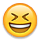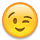MoneySavingExpert Chair, Martin Lewis · Editor, Marcus Herbert

# 'When would you use the geometric mean?' blog discussion.

edited 21 March 2011 at 12:24PM
33 replies 6.7K views
edited 21 March 2011 at 12:24PM
This is the discussion to link on the back of Martin's blog. Please read the blog first, as this discussion follows it.

## Replies

• Forumite
1.1K Posts✭✭✭
Can't see it being useful in any data set with a zero in anyway!
• Forumite
12.2K Posts✭✭✭✭✭
It's useful in any situation where you would expect exponential growth. E.g. the geometric mean of 1, 3 and 9 is 3; the geometric mean of 1, 10 and 100 is 10.

[Feel free to ignore this next sentance: It's the same as e to the arithmetic mean of the log of the numbers.]

There are times when I use it. Can't think of any examples right now, though.
• Forumite
380 Posts✭✭
When you want to shaft public sector workers, benefit claimants and pensioners by giving them smaller increases.
• Forumite
12.2K Posts✭✭✭✭✭
How about social skills of a group children?

I'm claiming that the difference between a 2 year old and a 4 year old is the same as the difference between a 4 year old and an 8 year old.
Therefore the average social skill level of a 2 year old and an 8 year old is that of a 4 year old. [The geometric average of 2 and 8 is 4.]
• Well according to the Wikipedia article, it's good when talking about growth. Choosing an appropriate theme of the day, gas prices, let's imagine prices increasing every year. Assuming you've managed thatlets imagine the amount it goes up is:

Year 1: 10%
Year 2: 12%
Year 3: 17%.

You then want to make a statement about the "average annual increase". The wikipedia article says that you're better off using the Geometric average rather than the Mean in this case. The reason is that you get an accurate calculation of what someone spending £100 in before the first increase would now have to pay once the final increase had been made. If you used the mean average, you wouldn't.

Calculations, the long winded year by year way:

Year 0: £100
Year 1: £110 (Year 0 + 10%)
Year 2: £123.20 (Year 1+ 12% )
Year 3: £144.14 ( Year 2 + 17 %)

The Mean average increase is (10+12+17) / 3 = 13%

Calculations using Mean average:

Year 0: £100
Year 1: £113 (Year 0 + 13%)
Year 2: £127.69 (Year 1 + 13%)
Year 3: £144.29 (Year 2 + 13%)

So using the Mean average you've overstated by 15p. This could be much larger with different figures for the interest.

Using the geometric average which is 12.96% you get:

Year 0: £100
Year 1: £112.96 (Year 0 + 12.96%)
Year 2: £127.60 (Year 1 + 12.96%)
Year 3: £144.14 (Year 2 + 12.96%)

Which is right for year 3 (what we wanted to find out) but wrong for years 1 and 2. Which we weren't bothered about. Also it won't help you with the total spend over the four years. Switch the scenario to savings and knowing year 3 is the only important factor as it says how much money you've got in your savings account.

Notes on calculating the geometric average.

Taking the cube root of (10*12*17) is wrong!. You must express the percentages as decimals. And since you're increasing them, you must add 1.

So the geometric average would be the cube root of (1.10*1.12*1.17).

So if you have a bunch of interest rates (APR's maybe) and you want to work out the effect of repeated compound interest then you can take the geometric average of the rates, and, assuming they are all cover the same time period (e.g. a year) then you can short cut doing multiple calculations with different amounts of money.
I generally post and run. You probably wont be able to engage me in conversation as I probably wont be around to read replies....
• Compound growth is probably the easiest place to understand the geometric mean.

Suppose a savings account pays compound interest and returns 5% in year 1, 10% in year 2 and 15% in year 3. An alternative savings account would need to pay compound interest at a rate of 9.92% per annum (ish) in order to provide the same return. A third savings account paying compound interest returning 10% per annum (The arithmetic mean) would yield a higher retun than both accounts. 9.92% is the geometric mean of 5%, 10% and 15%.

And since we're all in a mean mood: Suppose a rabbit runs across a field at a speed of 4mph. He / She then turns around and runs back across the same field at a speed of 2mph, covering the same distance over both journeys. What is the rabbit's overall average speed over the outward and return journeys? (Hint: It's not 3mph)
"Peter Pan is 2. Shirley Bassey is 3. Dr Ian Paisley is 4. King Lear is 5. Why?"

"...also known as taking in the Spanish Cub Scout leader. (Cryptic) (5)"

Thanks to MSE, I've seen Citizen Kane, 2001: A Space Odyssey and Serenity for FREE!• Seems to fail the common sense and simplicity test.

In the same way as 'seasonal adjustment' seems to make a nonsense of comparing house price statistics.

The problem in the rate of inflation has an enormous bearing on whether we are getting wealthier in real terms and the salaries, pensions and other benefits we receive or might expect to receive.

It does seem that in an era of large supermarkets, barcodes and computerised systems that it should be fairly straight forward to work out how much we are all paying on average for 100g of coffee, tea, chocolate or ipads and work out whether the cost of these is increasing or decreasing in a particular month.

Resorting to geometry that a money saving expert can't work out seems to suggest we are over complicating things?

R.
Smile, it makes people wonder what you have been up to.
• The 'classic' use for the geometric mean is where you're dealing with variables that have a multiplicative element.

An example is an investment of £100 which earns 10% the first year, 50% the second year, and 30% the third year, giving you £214.50 total.

Say you wanted to calculate the average rate of return...

The arithmetic mean would give the average rate of return as 30%, giving a total of £219.70, which is incorrect, as it ignores the fact that the increases are linked to each other as multiples (£100 x 1.1 x 1.5 x 1.3 = £214.50).

The geometric mean of 1.283(ish) is the correct 'average' multiple for each year that gets you to the correct end result.

This is presumably the logic that leads to it being used to assess inflation figures, which also have a multiplicative relationship with prices of previous years.

Edit: Looks like three or four of us all posted this usage at the same time.• Colxfile wrote: »
Suppose a rabbit runs across a field at a speed of 4mph. He / She then turns around and runs back across the same field at a speed of 2mph, covering the same distance over both journeys. What is the rabbit's overall average speed over the outward and return journeys? (Hint: It's not 3mph)

Are we talking speed (un-directional scalar quantity) or the directional vector 'velocity'?
• VT82 wrote: »
Can't see it being useful in any data set with a zero in anyway!

If used in calculations about growth, zero growth would be shown as a 1. If there was -100% growth, then you'd get a zero in the calculation, and it would still be correct. It would be the equivalent of saying "you've lost all your money". Any future proposed interest rates, applied to zero funds would come out as zero. Which would be correct. See my earlier post...
I generally post and run. You probably wont be able to engage me in conversation as I probably wont be around to read replies....
This discussion has been closed.
Latest MSE News and Guides